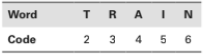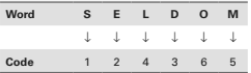# Mathematical Reasoning - Coding Decoding

>>>>>>>>Coding Decoding

• A

3456• B

3546• C

2345• D

2456• Option : A
• Explanation :These values have been assigned arbitrarily. The question can be solved on the basis of the relationship established. For RAIN, the code is 3456, so (a) is the answer.

• A

3 6 2 1• B

6 2 3 1• C

5 6 3 2• D

6 2 1 3• Option : C
• Explanation :On the basis of the analogical relationship established between the letters and the numbers, we can code ‘MODE’ as 5632.

• A

18−1−3−5• B

16−1−3−5• C

16−3−5−1• D

None of the above• A

20−26−7−22• B

20−26−6−22• C

26−20−7−22• D

26−20−22−7• A

13−18−8−13−5• B

13−17−6−12−4• C

12−17−7−12−5• D

None of the above• Option : A
• Explanation : The pattern of assignment is read as given in the following table.## Description

• Coding And Decoding Questions can be used to give quizzes by any candidate who is preparing for UGC NET Paper 1
• This Coding And Decoding Questions section will help you test your analytical skills in a tricky method, thereby giving you an edge over other students
• All candidates who have to appear for the Kendriya Vidyalaya Entrance exam can also refer to this mcq section.
• You can also get access to the Coding And Decoding MCQ ebook.
• Coding And Decoding Questions can be used in the preparation of JRF, CSIR, and various other exams.